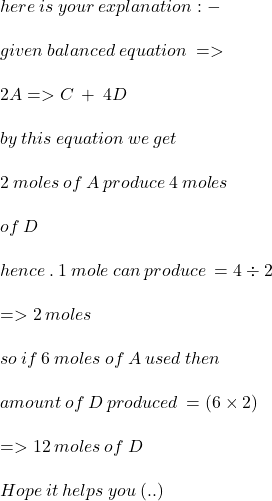Consider the balanced chemical equation below. 2 A ⟶ C + 4 D How many moles of D would be produced if 6 moles of A w

Question

Consider the balanced chemical equation below.

2 A ⟶ C + 4 D

How many moles of D would be produced if 6 moles of A were used?

in progress 0
7 months 2021-07-13T17:57:20+00:00 1 Answers 1 views 0